# Bayes theorem

### Home | Academic Articles

This is used to find conditional probabilities based on a weighted average.

What is a weighted average?

Example

For a store, 40% of the customers are male. The males spend \$274 on average while the females spend \$186 on average. What is the overall average spent?

If the gender split were 50/50, we could add \$274 and \$186 and divide the result by 2 to get \$230. Note that we could rewrite (274 + 186)/2 as (0.5)(274) + (0.5)(186). However, the gender split is 40/60. Thus, the answer is (0.4)(274) + (0.6)(186) = 221.20. Note that \$221.20 is closer to \$186 than \$274 since the majority of customers are female.

Multiplication Rule

We begin with the formula for conditional probability: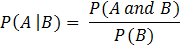Cross-multiplying, we get: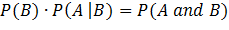This equation is used to find a weighted average.

Example

10% of people live in the target market

9% of those living in the target market respond to an offer

5% of those living outside the target market respond to an offer

What percentage of people overall respond to the offer?

To find the weighted average, we construct a tree.

P(TM) = 0.1            P(R | TM) = 0.09

P(TM and R) = (0.1)(0.09) = 0.009

P(Not TM) = 0.9     P(R | Not TM) = 0.05

P(Not TM and R) = (0.9)(0.05) = 0.045

P(R) = 0.009 + 0.045 = 0.054 = 5.4%

Some notes:

·        Since 10% live in the target market, that means that 90% live outside the target market. These two probabilities are the first terms on the left side of the multiplication rule for their respective branches.

·        The second term on the left side is the conditional probability of responding to the offer given that the person either lives in the target market or lives outside it.

·        When we multiply the two terms on the left side, we get the joint probability of the two events on the left side. When we multiply P(TM) and P(R | TM), we get P(TM and R).

·        The final percentage of those who respond at 5.4% is between P(R | TM) = 9% and P(R | not TM) = 5%. Note that the 5.4% is closer to 5% than 9% since the majority live outside the target market (90% versus 10%).

What percentage of those who respond to the offer live in the target market?

We want P(TM | R). In its infancy, Bayes theorem was known as inverse conditional probability. This is because a conditional probability, in this case P(R | TM), is used to construct a weighted average. We then use the weighted average to find the inverse conditional probability. For this problem, we can use the probabilities from the tree: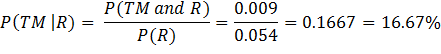Note that 16.67% is equivalent to 1 in 6. This is greater than the 10% who live in the target market. This is due to the fact that the response rate is higher in the target market.

Of those who do not respond, what percentage live in the target market?

In theory, we could construct another tree. However, we have enough information to construct a crosstab:

 TM Not TM Total R 0.009 0.045 0.054 Not R 0.091 0.855 0.946 Total 0.1 0.9 1.0

We want P(TM | not R).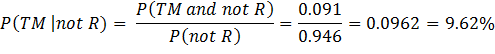Note that the 9.62% is less than the 10% who live in the target market. Again, this is due to the fact that the response rate is higher in the target market.

Example

A province is divided into three regions A, B and C. One-half live in A, one-third in B and one-sixth in C.

In the last election, the percentage in each district who voted was 50.2% in A, 57.3% in B and 60.9% in C.

Of those who voted, the percentage in each district who voted Liberal was 47.3% in A, 32.9% in B and 25.4% in C.

What percentage overall voted?

In this problem, the tree has 3 branches since there are 3 regions.

P(A) = 1 / 2           P(V | A)_= 0.502

P(A and V) = 0.502/2 = 0.251

P(B) = 1 /3            P(V | B) = 0.573

P(B and V) = 0.573/3 = 0.191

P(C) = 1 /6            P(V | B) = 0.609

P(B and V) = 0.609/6 = 0.1015

P(V) = 0.251 + 0.191 + 0.1015 = 0.5435 = 54.35%

Note that 54.35% is between the smallest voting percentage of 50.2% in region A and the largest voting percentage of 60.9% in region C.

Of those who voted, what percentage voted Liberal?

To solve this problem, the joint probabilities from the previous problem form the first terms of the left side of the multiplication rule.

P(A and V) = 0.251          P(L | A and V) = 0.473

P(A and V and L) = (0.251)(0.473) = 0.118723

P(B and V) = 0.191          P(L | B and V) = 0.329

P(B and V and L) = (0.191)(0.329) = 0.062839

P(C and V) = 0.1015        P(L | C and V) = 0.254

P(C and V and L) = (0.1015)(0.254) = 0.025781

P(V and L) = 0.118723 + 0.062839 + 0.025781 = 0.207343 = 20.7343%

Note that in constructing this tree, the joint probabilities have two common events, namely, that the person voted and that the person voted Liberal. The only event that varies is the region. Thus, when we add the probabilities, we get P(V and L).

However, 20.7343% is not the answer to the problem. This is the percentage of the entire population that voted Liberal which includes those who did not vote. We want P(L | V).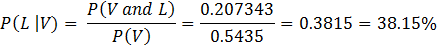Note that 38.15% is between the lowest percentage of 25.4% in region C and the highest percentage of 47.3% in region A.

Create a tree of voting patterns to determine the percentage of voters who did not vote Liberal.

P(V) = 0.5435        P(V and L) = 0.207343

P(V and not L) = 0.5435 – 0.207343 = 0.336157

P(not V) = 0.4565

Note that P(not V) is simply 1 – P(V) = 1 – 0.5435 = 0.4565. However, for this branch, this is the end of the line since it is nonsensical to speak of a person voting Liberal if the person did not vote. However, of those who did vote, we can divide them into those who voted Liberal and those who did not. We want P(not L | V).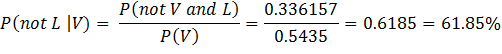Note that 38.15% and 61.85% sum to 100%.